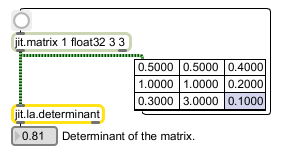# jit.la.determinant

Calculate the determinant of a matrix

## Description

The jit.la.determinant object calculates the determinant of a given input matrix, and send the result out the leftmost outlet. The input matrix must have typefloat32 or float64, and may have planecount 1 or 2. If the input matrix has a planecount of 2, it is assumed that the data is from the set of complex numbers, and the output determinant is a list of 2 floating point values. The first element is the real component and the second element is the imaginary component.

## Matrix Operator

matrix inputs:1, matrix outputs:0

## Attributes

Name Type g/s Description
thresh float The threshold value beneath which the absolute value of the result of internal calculations are considered to be equal to zero (default = 0.000000001)

## Examples